# Retinex图像增强算法

## Retinex图像增强算法

Retinex是一种常用的建立在科学实验和科学分析基础上的图像增强方法，它是Edwin.H.Land于1963年提出的。就跟Matlab是由Matrix和Laboratory合成的一样，Retinex也是由两个单词合成的一个词语，他们分别是retina 和cortex，即：视网膜和皮层。Land的retinex模式是建立在以下三个假设之上的：

• 真实世界是无颜色的，我们所感知的颜色是光与物质的相互作用的结果。我们见到的水是无色的，但是水膜—肥皂膜却是显现五彩缤纷，那是薄膜表面光干涉的结果。
• 每一颜色区域由给定波长的红、绿、蓝三原色构成的；
• 三原色决定了每个单位区域的颜色。

Retinex理论的基础理论是物体的颜色是由物体对长波（红色）、中波（绿色）、短波（蓝色）光线的反射能力来决定的，而不是由反射光强度的绝对值来决定的，物体的色彩不受光照非均匀性的影响，具有一致性，即retinex是以色感一致性（颜色恒常性）为基础的。不同于传统的线性、非线性的只能增强图像某一类特征的方法，Retinex可以在动态范围压缩、边缘增强和颜色恒常三个方面达到平衡，因此可以对各种不同类型的图像进行自适应的增强。

40多年来，研究人员模仿人类视觉系统发展了Retinex算法，从单尺度Retinex算法，改进成多尺度加权平均的MSR算法，再发展成彩色恢复多尺度MSRCR算法

## 一. 单尺度SSR(Single Scale Retinex)

### 1. 原理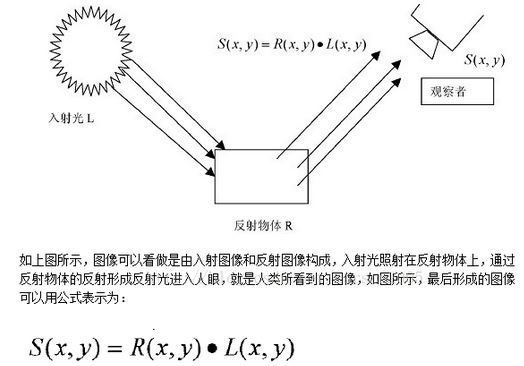I(x,y)=L(x,y)*R(x,y)　（乘，非卷积）　　(1-1)

式中： I(x,y)代表被观察或照相机接收到的图像信号；L(x,y)代表环境光的照射分量 ；R(x,y)表示携带图像细节信息的目标物体的反射分量 。

将(1-1)式两边取对数，则可抛开入射光的性质得到物体的本来面貌，即有关系式 ：

Log[R(x,y)] = Log[I(x,y)]-Log[L(x,y)];　　　　　　(1-2)

Log(R(x,y)）=(Log(I(x,y))-Log(I(x,y)*G(x,y)))  (1-3)

因此这个算法的细路就很简单了，具体步骤如下：

1、输入: 原始图像数据I(x,y),尺度（也就是所谓的模糊的半径）

2、处理：(1) 计算原始图像按指定尺度进行模糊后的图像 L(x,y);

(2) 按照2-2式的计算方法计算出 Log[R(x,y)]的值。

(3) 将 Log[R(x,y)]量化为0到255范围的像素值，作为最终的输出。

R(x,y) = ( Value - Min ) / (Max - Min) * (255-0)      (1-4)

### SSR:

#SSR

def replaceZeroes(data):
min_nonzero = min(data[nonzero(data)])
data[data == 0] = min_nonzero
return data

def SSR(img,size):
L_blur = cv2.GaussianBlur(img,(size,size),0)
eps = float(1e-10)

h,w = img.shape[:2]
dst_img = zeros((h,w),dtype = float32)
dst_Lblur = zeros((h, w),dtype=float32)
dst_R = zeros((h, w), dtype=float32)

img = replaceZeroes(img)
L_blur = replaceZeroes(L_blur)
cv2.log(img,dst_img)
cv2.log(L_blur,dst_Lblur)
log_R = cv2.subtract(dst_img,dst_Lblur)

cv2.normalize(log_R,dst_R,0,255,cv2.NORM_MINMAX)
log_uint8 = cv2.convertScaleAbs(dst_R)

minvalue,maxvalue,minloc,maxloc = cv2.minMaxLoc(log_R)
for i in range(h):
for j in range(w):
log_R[i,j] = (log_R[i,j]-minvalue)*255.0/(maxvalue-minvalue)
log_uint8 = cv2.convertScaleAbs(log_R)
return log_uint8

## 二. 多尺度MSR(Multi-Scale Retinex)

MSR是在SSR基础上发展来的，优点是可以同时保持图像高保真度与对图像的动态范围进行压缩的同时，MSR也可实现色彩增强、颜色恒常性、局部动态范围压缩、全局动态范围压缩，也可以用于X光图像增强。

（1）需要对原始图像进行每个尺度的高斯模糊，得到模糊后的图像Li(x,y),其中小标i表示尺度数。

(2) 对每个尺度下进行累加计算

Log[R(x,y)] =  Log[R(x,y)] + Weight(i)* ( Log[Ii(x,y)]-Log[Li(x,y)]);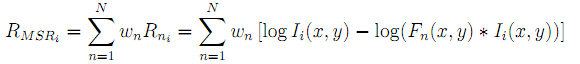### MSR

#MSR
def replaceZeroes(data):
min_nonzero = min(data[nonzero(data)])
data[data == 0] = min_nonzero
return data

def MSR(img, scales):
weight = 1/3.0
scales_size = 3

h, w = img.shape[:2]
dst_img = zeros((h, w), dtype=float32)
dst_Lblur = zeros((h, w), dtype=float32)
dst_R = zeros((h, w), dtype=float32)
log_R = zeros((h, w), dtype=float32)

for i in range(0,scales_size):
img = replaceZeroes(img)
L_blur = cv2.GaussianBlur(img, (scales[i], scales[i]), 0)
L_blur = replaceZeroes(L_blur)
cv2.log(img, dst_img)
cv2.log(L_blur, dst_Lblur)
log_R += weight*cv2.subtract(dst_img, dst_Lblur)

cv2.normalize(log_R, dst_R, 0, 255, cv2.NORM_MINMAX)
log_uint8 = cv2.convertScaleAbs(dst_R)

return log_uint8

## 三. 带色彩恢复的多尺度MSR(Multi-Scale Retinex with Color Restoration)

由于R是对数域的输出，要转换为数字图像，必须将他们量化为[0,255]的数字图像范畴，关于这个量化的算法，有这极为重要的意义，他的好坏直接决定了最终输出的图像的品质。

目前，结合上述文章中提出的一些过程，有4种方式进行处理：

第一种，也是最容易想到的就是，直接线性量化，即采用下式进行处理：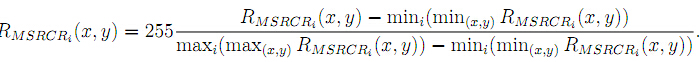这种方式，由于Retinex数据处理后的高动态特性，数据分布很广，会出现严重的两极化现象，一般难以获得满意的结果。

第二种，就是在经典的MSRCR文章《A Multiscale Retinex for Bridging the Gap Between Color Images and the Human Observation of Scenes》中提出的Canonical Gain/set 算法。计算公式如：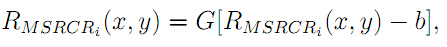其中G和b为经验参数。

第三种，实在上述文章中提到的Simplest Color Balance（我简写为SCR）方式，这种方式的处理类似于Photoshop中的自动色阶，他把数据按照一定的百分比去除最小和最大的部分，然后中间的部分重新线性量化到0和255之间。

第四种，就是GIMP的Retinex算法，这个可详见 带色彩恢复的多尺度视网膜增强算法（MSRCR）的原理、实现及应用 一文的描述。

还有一种方式，就是大家知道HDR的过程吧，他也是将高动态的数据量化到图像的可视范围，因此可以直接将这类算法应用与这个问题上。我也做了实验，效果似乎一般。

在用第二种或第三种方式处理时，最好还需要有个Color Restoration的过程，因为如果直接对MSR处理的结果进行量化，得到的图像往往整体偏灰度，这是由于原始的彩色值经过log处理后的数据范围就比较小了，这样各通道之间的差异也很小，而之后的线性量化比log曲线要平滑很多，因此整体就丧失了彩色。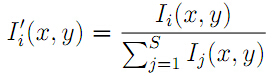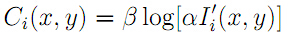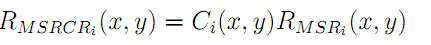其中β=46，α=125为经验参数，但是最终我的分析认为β不可能取这么大，取1试验表明效果还不错。

对于一些原始图像HUE较为合理的图，如果用经典的MSRCR算法，会导致处理后的图容易偏色，上述论文提出了对图像的Intensity数据进行Retinex处理，然后再把数据根据原始的RGB的比例映射到每个通道，这样就能在保留原始颜色分布的基础上增强图像，文章中称其为MSRCP。

MSRCR算法步骤：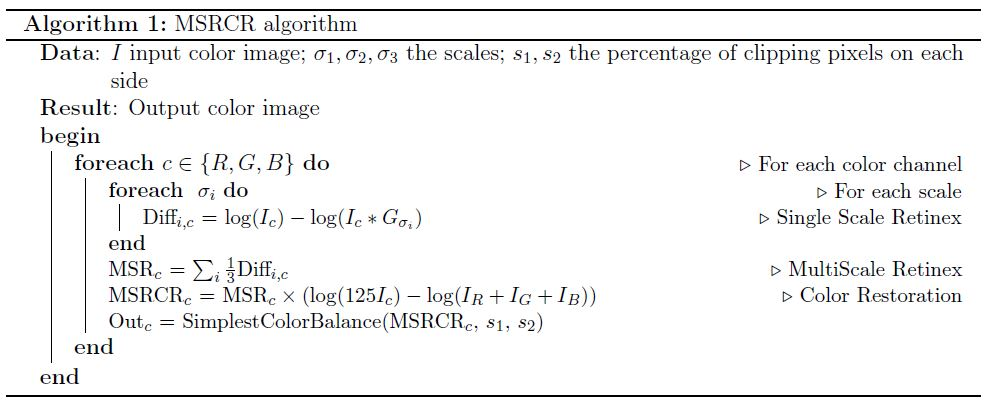MSRCR实现

#MSRCR
def replaceZeroes(data):
min_nonzero = min(data[nonzero(data)])
data[data == 0] = min_nonzero
return data

#simple color balance
def simple_color_balance(input_img,out_img,s1,s2):
h,w = input_img.shape[:2]
sort_img = input_img.copy()
one_dim_array = sort_img.flatten()#转化为一维数组
sort_array = sorted(one_dim_array)#对一维数组按升序排序

per1 = int((h*w)*s1/100)
minvalue = sort_array[per1]

per2 = int((h*w)*s2/100)
maxvalue = sort_array[(h*w)-1-per2]

#实施简单白平衡算法
if(maxvalue<=minvalue):
for i in range(h):
for j in range(w):
out_img[i,j] = maxvalue
else:
scale = 255.0/(maxvalue-minvalue)
for m in range(h):
for n in range(w):
if(input_img[m,n] < minvalue):
out_img[m,n] = 0
elif(input_img[m,n] > maxvalue):
out_img[m, n] = 255
else:
out_img[m, n] = scale*(input_img[m,n]-minvalue)#映射中间段的图像像素

out_img= cv2.convertScaleAbs(out_img)

def MSRCR(img,MSRCR_Out,scles,s1,s2):
h,w = img.shape[:2]
scles_size = 3
epc = float(1e-4)
B = img[:,:,0]
G = img[:, :, 1]
R = img[:, :, 2]

dst_img = zeros((h, w), dtype=float32)
dst_Lblur = zeros((h, w), dtype=float32)
log_R = zeros((h, w), dtype=float32)
I =  zeros((h, w), dtype=float32)

I = (B+G+R)
I = replaceZeroes(I)

for j in range(3):
img[:, :, j] = replaceZeroes(img[:, :, j])
for i in range(0, scles_size):
L_blur = cv2.GaussianBlur(img[:, :, j], (scales[i], scales[i]), 0)
L_blur = replaceZeroes(L_blur)
cv2.log(img[:, :, j], dst_img)
cv2.log(L_blur, dst_Lblur)
log_R += cv2.subtract(dst_img, dst_Lblur)
MSR = log_R / 3.0
MSRCR = MSR*(cv2.log(125.0*img[:, :, j]) - cv2.log(I))
simple_color_balance(MSRCR,MSRCR_Out[:,:,j],s1,s2)


MSRCP算法：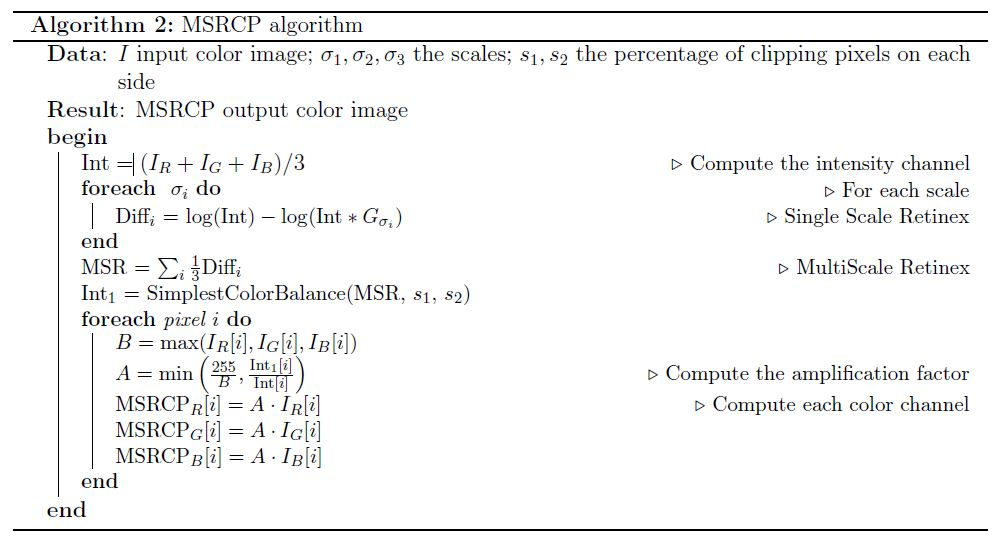MSRCP实现：

##MSRCP
def replaceZeroes(data):#这个函数主要是防止取对数的时候，出现去穷小的结果
min_nonzero = min(data[nonzero(data)])
data[data == 0] = min_nonzero
return data

#simple color balance
def simple_color_balance(input_img,out_img,s1,s2):
h,w = input_img.shape[:2]
sort_img = input_img.copy()
one_dim_array = sort_img.flatten()#转化为一维数组
sort_array = sorted(one_dim_array)#对一维数组按升序排序

per1 = int((h*w)*s1/100)
minvalue = sort_array[per1]

per2 = int((h*w)*s2/100)
maxvalue = sort_array[(h*w)-1-per2]

#实施简单白平衡算法
if(maxvalue<=minvalue):
for i in range(h):
for j in range(w):
out_img[i,j] = maxvalue
else:
scale = 255.0/(maxvalue-minvalue)
for m in range(h):
for n in range(w):
if(input_img[m,n] < minvalue):
out_img[m,n] = 0
elif(input_img[m,n] > maxvalue):
out_img[m, n] = 255
else:
out_img[m, n] = scale*(input_img[m,n]-minvalue)#映射中间段的图像像素

def MSRCP(img,scles,s1,s2):
h,w = img.shape[:2]
scles_size = 3
epc = float(1e-4)
B_chan = img[:,:,0]
G_chan = img[:, :, 1]
R_chan = img[:, :, 2]

dst_img = zeros((h, w), dtype=float32)
dst_Lblur = zeros((h, w), dtype=float32)
log_R = zeros((h, w), dtype=float32)
I =  zeros((h, w), dtype=float32)
Int1 = zeros((h, w), dtype=float32)
R_channel_out = zeros((h, w), dtype=float32)
G_channel_out = zeros((h, w), dtype=float32)
B_channel_out = zeros((h, w), dtype=float32)
MSRCP_Out_img = zeros(img.shape, dtype=float32)
MSRCP_Out = zeros(img.shape, dtype=uint8)

I = (B_chan+G_chan+R_chan)/3.0
I = replaceZeroes(I)

for i in range(0, scles_size):
L_blur = cv2.GaussianBlur(I, (scales[i], scales[i]), 0)
L_blur = replaceZeroes(L_blur)
cv2.log(I, dst_img)
cv2.log(L_blur, dst_Lblur)
log_R += (dst_img - dst_Lblur)
MSR = log_R / 3.0
simple_color_balance(MSR,Int1,s1,s2)

for i in range(h):
for j in range(w):
maxtmp = max(B_chan[i,j],G_chan[i,j])
B = max(maxtmp,R_chan[i,j])
if(I[i,j] == 0):
I[i, j] = 1
A = min(255/B,Int1[i,j]/I[i,j])
R_channel_out[i,j] = A*R_chan[i,j]
G_channel_out[i, j] = A * G_chan[i, j]
B_channel_out[i, j] = A * B_chan[i, j]

MSRCP_Out_img = cv2.merge([B_channel_out,G_channel_out,R_channel_out])
MSRCP_Out = cv2.convertScaleAbs(MSRCP_Out_img)

return MSRCP_Out

### 四. 图像效果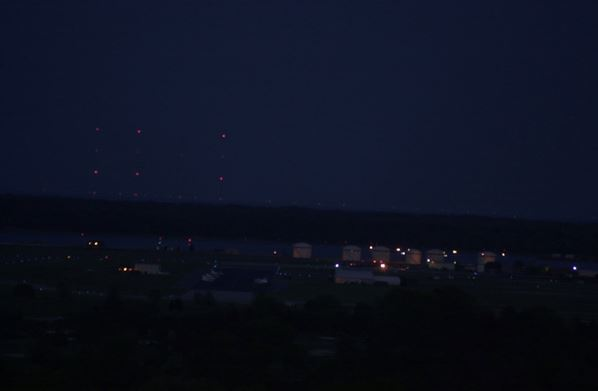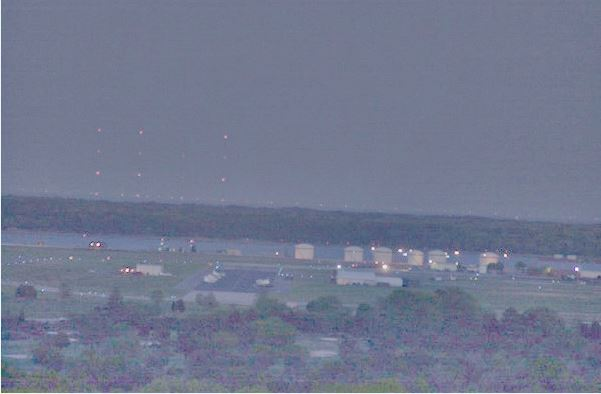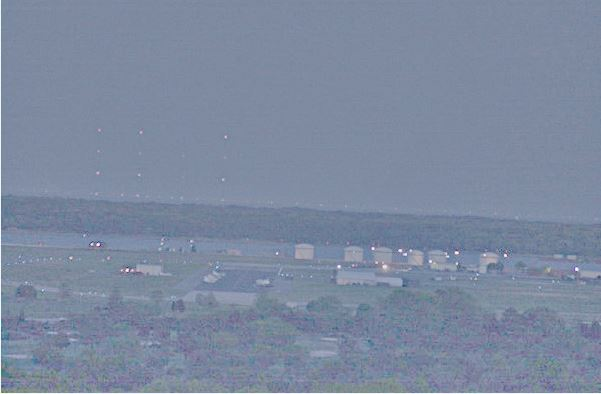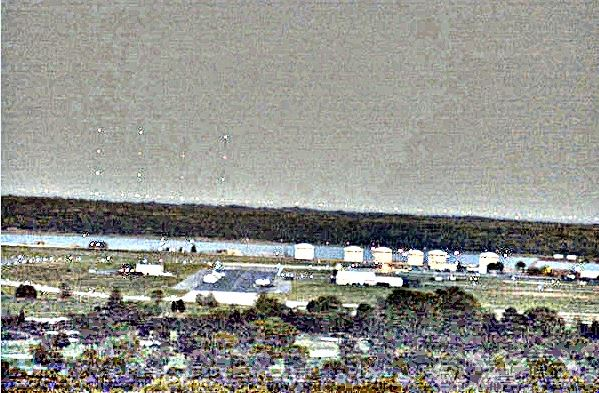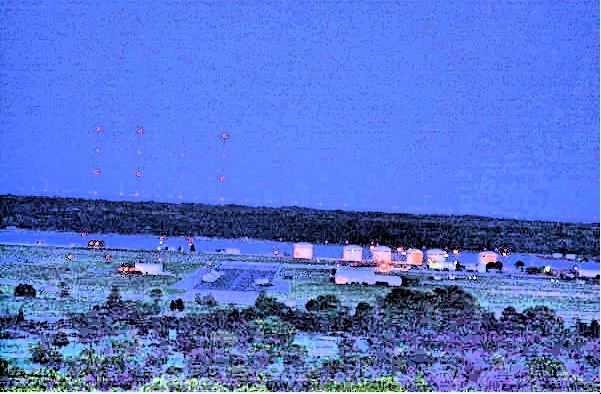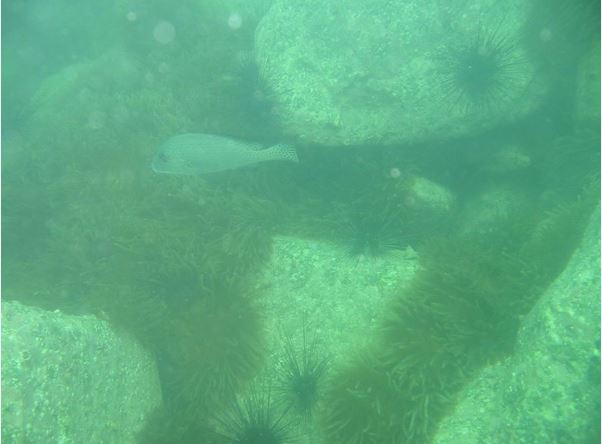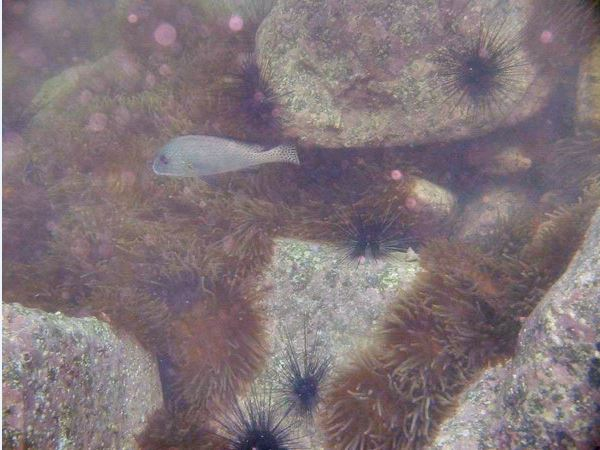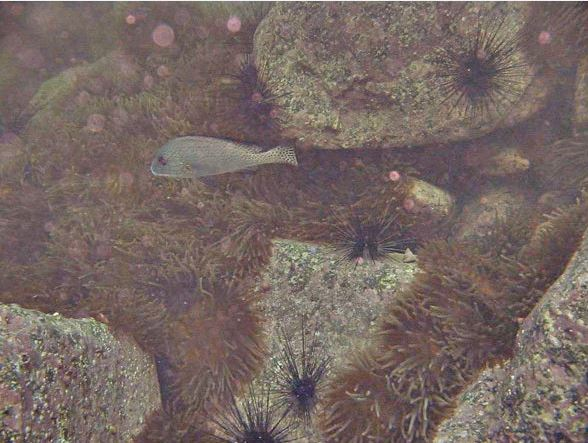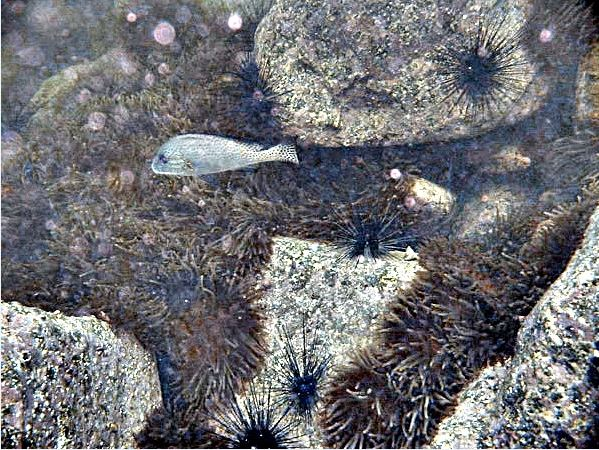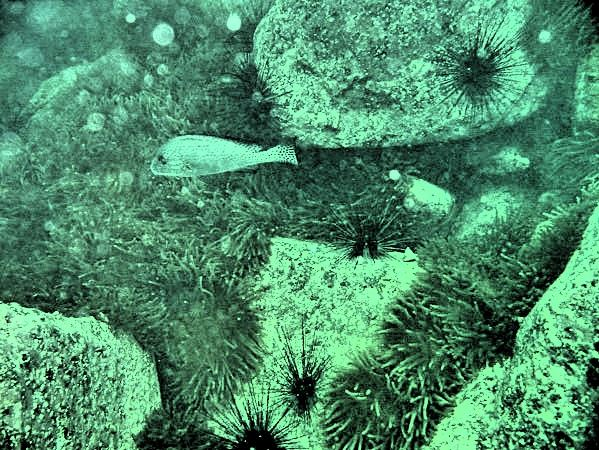从上到下分别是原始图、SSR、MSR、MSRCR、MSRCP，其中多尺度图像中的尺度因子都是15,101,301

参考文献：

Retinex图像增强算法(SSR, MSR, MSRCR)详解及其OpenCV源码

msrcr(Multi-Scale Retinex with Color Restoration) 带色彩恢复的多尺度视网膜增强算法 整理

Multiscale Retinex

11-23
07-214万+09-156066
12-132764
10-232881
08-124350
07-267万+
07-114万+